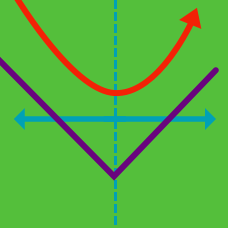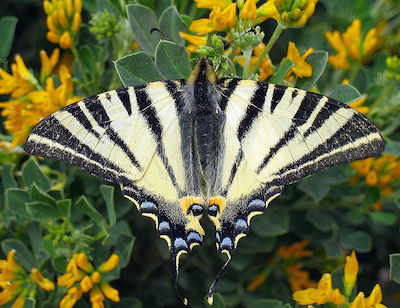Quantitative Finance

# Option Strategies: Level 2 Challenges

Let Y be the strangle that consists of the $45 put and the$55 call. An investor sold the strangle for \$5. What is the range of values of the stock on expiration, such that the investor would have made money?You are long a 5-10-15 butterfly. On which of the following expiration price(s) will you make the most money?

Which of the following is a definite benefit of owning a call spread rather than an outright call?

The ATM straddle with 5 days to expiry currently has a theta value of 12.

Assuming constant volatility in the term structure, what is the approximate price of the ATM straddle with 20 days to expiry?

True, False or It depends?

We know that the ATM straddle price can be approximated by the formula

$Y_{ATM} = \frac{ 1}{ 2000} S \sigma \sqrt{t}.$

Since gamma is the second (partial) derivative with respect to the underlying price $$S$$, the gamma of the straddle is 0.

×